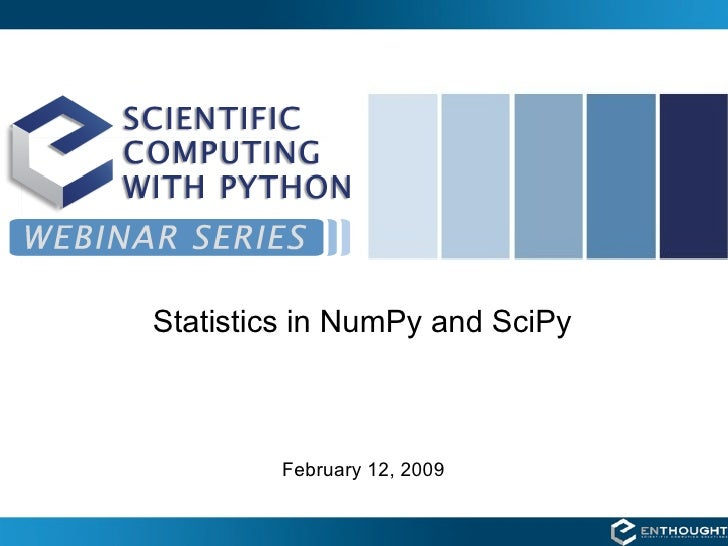Successfully reported this slideshow.Upcoming SlideShare
×

# NumPy/SciPy Statistics

23,417 views

Published on

Travis Oliphant, author of NumPy, presents an introduction into NumPy and SciPy tools for statistical analysis including scipy.stats.

Published in: Technology
• Full Name
Comment goes here.

Are you sure you want to Yes No• The #1 Woodworking Resource With Over 16,000 Plans, Download 50 FREE Plans... ■■■ http://t.cn/A6hKwqcb

Are you sure you want to  Yes  No

Are you sure you want to  Yes  No

Are you sure you want to  Yes  No
• Is there a PDF of this presentation available?

Are you sure you want to  Yes  No

Are you sure you want to  Yes  No

### NumPy/SciPy Statistics

1. 1. Statistics in NumPy and SciPy February 12, 2009
2. 2. Enthought Python Distribution (EPD) MORE THAN SIXTY INTEGRATED PACKAGES • Python 2.6 • Repository access • Science (NumPy, SciPy, etc.) • Data Storage (HDF, NetCDF, etc.) • Plotting (Chaco, Matplotlib) • Networking (twisted) • Visualization (VTK, Mayavi) • User Interface (wxPython, Traits UI) • Multi-language Integration • Enthought Tool Suite (SWIG,Pyrex, f2py, weave) (Application Development Tools)
3. 3. Enthought Training Courses Python Basics, NumPy, SciPy, Matplotlib, Chaco, Traits, TraitsUI, …
4. 4. PyCon http://us.pycon.org/2010/tutorials/ Introduction to Traits Corran Webster
5. 5. Upcoming Training Classes March 1 – 5, 2009 Python for Scientists and Engineers Austin, Texas, USA March 8 – 12, 2009 Python for Quants London, UK http://www.enthought.com/training/
6. 6. NumPy / SciPy Statistics
7. 7. Statistics overview • NumPy methods and functions – .mean, .std, .var, .min, .max, .argmax, .argmin – median, nanargmax, nanargmin, nanmax, nanmin, nansum • NumPy random number generators • Distribution objects in SciPy (scipy.stats) • Many functions in SciPy – f_oneway, bayes_mvs – nanmedian, nanstd, nanmean
8. 8. NumPy methods • All array objects have some “statistical” methods – .mean(), .std(), .var(), .max(), .min(), .argmax(), .argmin() – Take an axis keyword that allows them to work on N-d arrays (shown with .sum). axis=0 axis=1
9. 9. NumPy functions • median • nan-functions (ignore nans) – nanmax – nanmin – nanargmin – nanargmax – nansum • Can also use masks and regular functions
10. 10. NumPy Random Number Generators • Based on Mersenne twister algorithm • Written using PyRex / Cython • Univariate (over 40) • Multivariate (only 3) – multinomial – dirichlet – multivariate_normal • Convenience functions – rand, randn, randint, ranf
11. 11. Statistics scipy.stats — CONTINUOUS DISTRIBUTIONS over 80 continuous distributions! METHODS pdf entropy cdf nnlf rvs moment ppf freeze stats fit sf isf
12. 12. Using stats objects DISTRIBUTIONS >>> from scipy.stats import norm # Sample normal dist. 100 times. >>> samp = norm.rvs(size=100) >>> x = linspace(-5, 5, 100) # Calculate probability dist. >>> pdf = norm.pdf(x) # Calculate cummulative Dist. >>> cdf = norm.cdf(x) # Calculate Percent Point Function >>> ppf = norm.ppf(x)
13. 13. Distribution objects Every distribution can be modified by loc and scale keywords (many distributions also have required shape arguments to select from a family) LOCATION (loc) --- shift left (<0) or right (>0) the distribution SCALE (scale) --- stretch (>1) or compress (<1) the distribution
14. 14. Example distributions NORM (norm) – N(µ,σ) Only location and scale location mean µ arguments: scale standard deviation σ LOG NORMAL (lognorm) log(S) is N(µ, σ) location offset from zero (rarely used) S is lognormal scale eµ one shape parameter! shape σ
15. 15. Setting location and Scale NORMAL DISTRIBUTION >>> from scipy.stats import norm # Normal dist with mean=10 and std=2 >>> dist = norm(loc=10, scale=2) >>> x = linspace(-5, 15, 100) # Calculate probability dist. >>> pdf = dist.pdf(x) # Calculate cummulative dist. >>> cdf = dist.cdf(x) # Get 100 random samples from dist. >>> samp = dist.rvs(size=100) # Estimate parameters from data >>> mu, sigma = norm.fit(samp) .fit returns best >>> print “%4.2f, %4.2f” % (mu, sigma) shape + (loc, scale) 10.07, 1.95 that explains the data
16. 16. Statistics scipy.stats — Discrete Distributions 10 standard discrete distributions (plus any finite RV) METHODS pmf moment cdf entropy rvs freeze ppf stats sf isf
17. 17. Using stats objects CREATING NEW DISCRETE DISTRIBUTIONS # Create loaded dice. >>> from scipy.stats import rv_discrete >>> xk = [1,2,3,4,5,6] >>> pk = [0.3,0.35,0.25,0.05, 0.025,0.025] >>> new = rv_discrete(name='loaded', values=(xk,pk)) # Calculate histogram >>> samples = new.rvs(size=1000) >>> bins=linspace(0.5,5.5,6) >>> subplot(211) >>> hist(samples,bins=bins,normed=True) # Calculate pmf >>> x = range(0,8) >>> subplot(212) >>> stem(x,new.pmf(x))
18. 18. Statistics CONTINUOUS DISTRIBUTION ESTIMATION USING GAUSSIAN KERNELS # Sample two normal distributions # and create a bi-modal distribution >>> rv1 = stats.norm() >>> rv2 = stats.norm(2.0,0.8) >>> samples = hstack([rv1.rvs(size=100), rv2.rvs(size=100)]) # Use a Gaussian kernel density to # estimate the PDF for the samples. >>> from scipy.stats.kde import gaussian_kde >>> approximate_pdf = gaussian_kde(samples) >>> x = linspace(-3,6,200) # Compare the histogram of the samples to # the PDF approximation. >>> hist(samples, bins=25, normed=True) >>> plot(x, approximate_pdf(x),'r')
19. 19. Other functions in scipy.stats • Statistical Tests (Anderson, Wilcox, etc.) • Other calculations (hmean, nanmedian) • Work in progress • A great place to jump in and help
20. 20. Other statistical Resources • scikits.statsmodels • RPy2 • PyMC